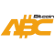Bitcoin ABC  0.22.13 P2P Digital Currency
aserti32d_tests.cpp File Reference
`#include <pow/aserti32d.h>`
`#include <chain.h>`
`#include <chainparams.h>`
`#include <config.h>`
`#include <consensus/activation.h>`
`#include <pow/pow.h>`
`#include <test/util/setup_common.h>`
`#include <boost/test/unit_test.hpp>`
`#include <cmath>`
Include dependency graph for aserti32d_tests.cpp:

Go to the source code of this file.

## Classes

class  ChainParamsWithDAAActivation

## Functions

static CBlockIndex GetBlockIndex (CBlockIndex *pindexPrev, int64_t nTimeInterval, uint32_t nBits)

static double TargetFromBits (const uint32_t nBits)

static double GetASERTApproximationError (const CBlockIndex *pindexPrev, const uint32_t finalBits, const CBlockIndex *pindexAnchorBlock)

BOOST_AUTO_TEST_CASE (asert_difficulty_test)

static std::string StrPrintCalcArgs (const arith_uint256 refTarget, const int64_t targetSpacing, const int64_t timeDiff, const int64_t heightDiff, const arith_uint256 expectedTarget, const uint32_t expectednBits)

BOOST_AUTO_TEST_CASE (calculate_asert_test)

BOOST_AUTO_TEST_CASE (asert_activation_anchor_test)
Test transition of cw144 to ASERT algorithm, which involves the selection of an anchor block. More...

## ◆ BOOST_AUTO_TEST_CASE() [1/3]

 BOOST_AUTO_TEST_CASE ( asert_difficulty_test )

Definition at line 58 of file aserti32d_tests.cpp.

Here is the call graph for this function:

## ◆ BOOST_AUTO_TEST_CASE() [2/3]

 BOOST_AUTO_TEST_CASE ( calculate_asert_test )

Definition at line 370 of file aserti32d_tests.cpp.

Here is the call graph for this function:

## ◆ BOOST_AUTO_TEST_CASE() [3/3]

 BOOST_AUTO_TEST_CASE ( asert_activation_anchor_test )

Test transition of cw144 to ASERT algorithm, which involves the selection of an anchor block.

Now we'll try adding on blocks to activate ASERT. The activation block is going to be our anchor block. We will make several distinct anchor blocks.

Definition at line 547 of file aserti32d_tests.cpp.

Here is the call graph for this function:

## ◆ GetASERTApproximationError()

 static double GetASERTApproximationError ( const CBlockIndex * pindexPrev, const uint32_t finalBits, const CBlockIndex * pindexAnchorBlock )
static

Definition at line 38 of file aserti32d_tests.cpp.

Here is the call graph for this function:
Here is the caller graph for this function:

## ◆ GetBlockIndex()

 static CBlockIndex GetBlockIndex ( CBlockIndex * pindexPrev, int64_t nTimeInterval, uint32_t nBits )
static

Definition at line 21 of file aserti32d_tests.cpp.

Here is the call graph for this function:
Here is the caller graph for this function:

## ◆ StrPrintCalcArgs()

 static std::string StrPrintCalcArgs ( const arith_uint256 refTarget, const int64_t targetSpacing, const int64_t timeDiff, const int64_t heightDiff, const arith_uint256 expectedTarget, const uint32_t expectednBits )
static

Definition at line 352 of file aserti32d_tests.cpp.

Here is the call graph for this function:
Here is the caller graph for this function:

## ◆ TargetFromBits()

 static double TargetFromBits ( const uint32_t nBits )
static

Definition at line 34 of file aserti32d_tests.cpp.

Here is the caller graph for this function: